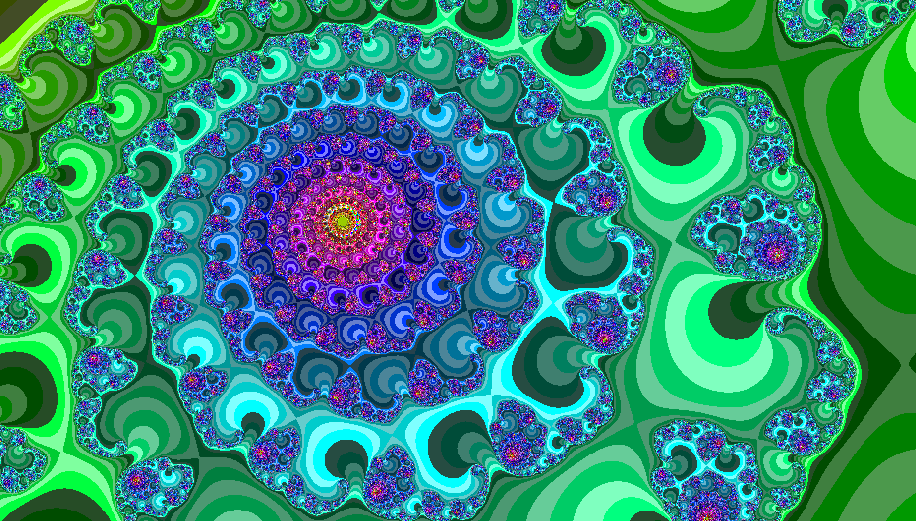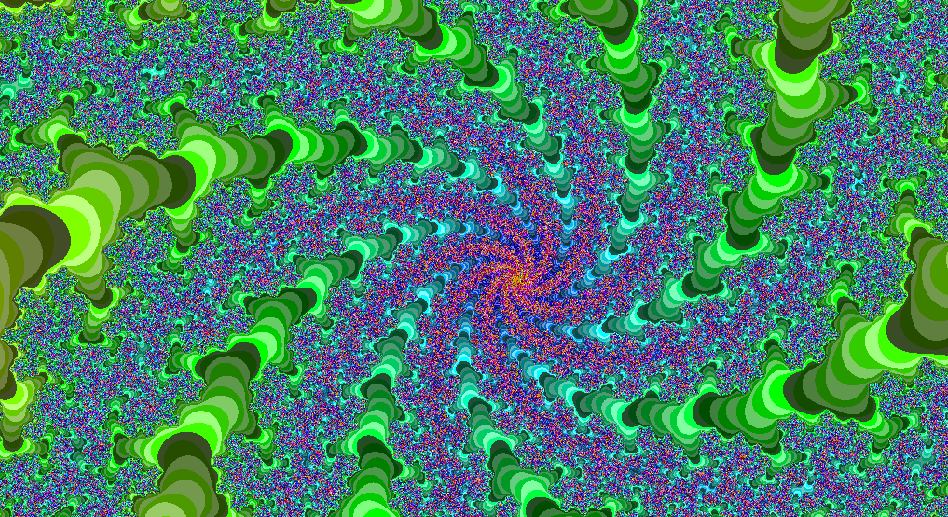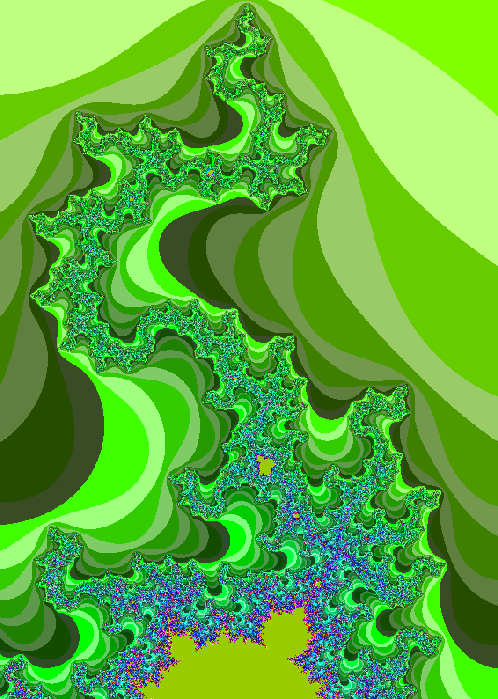# Fractals

See also Sierpinski Triangle, Koch Snowflake & Iterated Function Systems.

For me, this is a truly fascinating area of Mathematics since it is astounding that an object of such overwhelmingly infinite complexity may be generated from iterations of such simple equations.

## Julia Fractal

I look at the behaviour of the polynomial:In which z is a complex number (taking the form: z = u+iv where i is the square root of -1); and c is a complex constant, commonly known as the Julia constant.

To generate the fractal image, I plot complex values on the XY Plane. The Real part of z spans the X-axis, and similarly the Imaginary part spans the Y-axis. In this way the Real XY plane can be visualised as the Argand (or Complex) plane.

To plot the fractal, I take a portion of the Complex plane (the image size) and divide it up into a few hundred thousand discrete points (the image resolution); I then proceed to process each point to determine the colour it should display. The algorithm to determine such colour is as follows

• Take a point z in the complex plane, calculate f (z) for a predetermined value of the Julia Constant.
• Take the result of the above calculation and recursively apply the above function to obtain f ( f (z)).
• Count the number of iterations taken for either the norm (magnitude) of the resultant complex number to exceed a certain value (in this case: 2), or for the number of iterations to exceed an iteration limit (in this case: 255).
• The recorded number of iterations is then the colour of the point z.
• Repeat the above procedure for every point in the plane (in this case, every point in the image of the specified size & resolution).

### Julia Fractal Generating Function

Select all
```;;-----------------=={ Julia Set Fractal }==------------------;;
;;                                                            ;;
;;  The function will create a representation of the Julia    ;;
;;  set by iteration of the function:                         ;;
;;                                                            ;;
;;    f(z) = z^2 + c                                          ;;
;;                                                            ;;
;;  Where 'z' & 'c' are complex numbers. The fractal colour   ;;
;;  is determined by the number of iterations (less than a    ;;
;;  predetermined maximum iteration number) before the        ;;
;;  norm of the complex number generated by the above         ;;
;;  function reaches a limit.                                 ;;
;;                                                            ;;
;;  'c' is the constant for each calculation, this            ;;
;;  distinguishes the Julia set from the Mandelbrot set       ;;
;;  (in which 'c' is set to the complex values of each point) ;;
;;  For this reason, there are infinitely many Julia fractals,;;
;;  but only one Mandelbrot fractal.                          ;;
;;                                                            ;;
;;  More interestingly, there exists a Julia fractal for      ;;
;;  every point of the Mandelbrot fractal.                    ;;
;;------------------------------------------------------------;;
;;  Note:                                                     ;;
;;  --------                                                  ;;
;;  Fractal calculation is CPU intensive and may take a       ;;
;;  long time to generate the result. Reduce the iteration    ;;
;;  limit and image size & resolution to decrease calculation ;;
;;  time.                                                     ;;
;;------------------------------------------------------------;;
;;  Author: Lee Mac, Copyright © 2011 - www.lee-mac.com       ;;
;;------------------------------------------------------------;;

(defun c:jfract ( / a b c i im[c] lim re[c] res x xmax xmin y ymax ymin )
(setvar 'PDMODE 0)
(setq
lim 255     ;; Iteration Limit

xmin -0.60  ;; }
xmax -0.30  ;; | Image
ymin -0.35  ;; | Size
ymax -0.05  ;; }

res 0.0025  ;; Image Resolution

Re[c] 0.285 ;; Real Coefficient of Julia Constant
Im[c] 0.01  ;; Imaginary Coefficient of Julia Constant
)
(setq x (- xmin res))
(while (<= (setq x (+ x res)) xmax)
(setq y (- ymin res))
(while (<= (setq y (+ y res)) ymax)
(setq
i 0
a x
b y
)
(while
(and
(< (+ (* a a) (* b b)) 4.)
(<= (setq i (1+ i)) lim)
)
(setq
c (* 2.0 a b)
a (+ (- (* a a) (* b b)) Re[c])
b (+ c Im[c])
)
)
(entmake
(list
'(0 . "POINT")
(list 10 x y)
(cons 62 (1+ (rem (+ 30 i) 255)))
)
)
)
)
(princ)
)
```

### Examples of Julia Fractals## Mandelbrot Fractal

The Mandelbrot Fractal is generated using the same function and algorithm as the Julia Fractal, however, the value of the previous Julia Constant in the above calculations becomes the point being processed, and the value of z is initially zero for every calculation

In this way we are effectively calculating the set of points c for which the sequence obtained after applying the function f recursively, does not diverge.

It is interesting to note that there exists only one Mandelbrot Fractal, but infinitely many Julia Fractals; furthermore, there exists a Julia Fractal at every point in the Mandelbrot set.

### Mandelbrot Fractal Generating Function

Select all
```;;--------------=={ Mandelbrot Set Fractal }==----------------;;
;;                                                            ;;
;;  The function will create a representation of the          ;;
;;  Mandelbrot set by iteration of the function:              ;;
;;                                                            ;;
;;    f(z) = z^2 + c                                          ;;
;;                                                            ;;
;;  Where 'z' & 'c' are complex numbers. The fractal colour   ;;
;;  is determined by the number of iterations (less than a    ;;
;;  predetermined maximum iteration number) before the        ;;
;;  norm of the complex number generated by the above         ;;
;;  function reaches a limit.                                 ;;
;;                                                            ;;
;;  'c' is a point in the complex plane. This differs from    ;;
;;  the Julia set in that each point in the calculation of    ;;
;;  the Mandelbrot set is effectively a Julia constant.       ;;
;;  This means that a Julia fractal may be generated from     ;;
;;  every point in the Mandelbrot set...                      ;;
;;------------------------------------------------------------;;
;;  Note:                                                     ;;
;;  --------                                                  ;;
;;  Fractal calculation is CPU intensive and may take a       ;;
;;  long time to generate the result. Reduce the iteration    ;;
;;  limit and image size & resolution to decrease calculation ;;
;;  time.                                                     ;;
;;------------------------------------------------------------;;
;;  Author: Lee Mac, Copyright © 2011 - www.lee-mac.com       ;;
;;------------------------------------------------------------;;

(defun c:mfract ( / a b c i lim res x xmax xmin y ymax ymin )
(setvar 'PDMODE 0)
(setq
lim 255     ;; Iteration Limit

xmin -2.0  ;; }
xmax  0.7  ;; | Image
ymin -1.2  ;; | Size
ymax  1.2  ;; }

res 0.02   ;; Image Resolution
)
(setq x (- xmin res))
(while (<= (setq x (+ x res)) xmax)
(setq y (- ymin res))
(while (<= (setq y (+ y res)) ymax)
(setq
i 0
a 0.0
b 0.0
)
(while
(and
(< (+ (* a a) (* b b)) 4.)
(<= (setq i (1+ i)) lim)
)
(setq
c (* 2.0 a b)
a (+ (- (* a a) (* b b)) x)
b (+ c y)
)
)
(entmake
(list
'(0 . "POINT")
(list 10 x y)
(cons 62 (1+ (rem (+ 70 i) 255)))
)
)
)
)
(princ)
)
```

### Mandelbrot FractalSee also Sierpinski Triangle, Koch Snowflake & Iterated Function Systems.

### Instructions for Running

Please refer to How to Run an AutoLISP Program.

Note: Fractal calculation is extremely CPU intensive involving repeated calculations up to a limit and the creation of coloured point entities for every pixel under the resolution specified. As a result, this process may take a long time to generate the result; reduce the iteration limit and image size and resolution to decrease calculation times.

textsize